The breakeven formula is used to calculate the point at which revenues equal costs, known as the breakeven point. It is the point at which a business “breaks even,” or recovers the amount of money that has been put into the business.

Remember
The breakeven point is the point at which a business may begin to turn a profit. Up until this point, costs have outpaced revenues.

Because profit is essentially surplus revenue, there has not (and cannot) be any profit while costs continue to exceed revenues. At the breakeven point revenues equal costs. There is no surplus revenue, so there is also no profit, but sales after the breakeven point will generate profit. Keep in mind, the breakeven point is by no means a guaranteed milestone for any business.

To accurately calculate the breakeven point of a venture (or a product line, or a business unit, etc.) you must have a complete picture of both fixed costs and variable costs.

Fixed Costs Fixed costs are costs that do not change with the number of units produced or sold—things like rent, salaries, or insurance. For the breakeven calculation, use a total of all fixed costs.

Variable Costs Variable costs are costs that do change with the number of units produced or sold. For businesses that offer services instead of physical products, variable costs are costs that change with the number of services executed. These costs include production materials, components, supplies, and other consumables directly tied to production or service execution. For the breakeven calculation, variable costs are the total costs on a per-unit basis.

To determine the breakeven point in units, use the following formula:Where: Fixed costs are the total fixed costs for the period The price is the per-unit selling price Variable costs are the total per-unit cost

The denominator of the breakeven equation is known as the contribution margin, or the amount of money available from each sale that is left over to pay the company’s fixed costs. Let’s take a look at an example of the breakeven formula in action.

## For Example – The Breakeven Equation In Action

An online retailer adds up her fixed costs including hosting for her online store and rent and utilities on the space she uses to store her inventory. Total fixed costs for an average month come to \$3,980. Variable costs, including the cost to purchase and ship the products she sells, total \$7.46 per unit, and she wants to sell them for \$13.99 each. With our values plugged into the formula, the breakeven calculation appears as follows:Following the order of operations and solving the contribution margin in the equation’s denominator, we are left with the following formula that is easily solved.Because our fixed costs were totaled for the period of a single month, we can see that our online retailer would have to sell 610 units (no one is interested in purchasing half a unit) in a month to break even. If up until now sales averaged four hundred units per month, that is a worrisome sign. By substituting different numbers for each of the pieces of the equation—fixed costs, variable costs, and sales price—it is possible to model the effects of different strategic options. What would happen if you were able to cut your variable costs by even just nine cents per unit? How much lower would the sales threshold be before you were able to break even in a month, a quarter, or a year? If you are considering lowering your sales price, how many more units would you have to sell in a period to cover your fixed costs? Simply swap out the numbers in the equation and you will have your answer.

Question What if I want to determine the number of sales dollars I will have to reach to break even instead of expressing that threshold in units?

To uncover the breakeven point in dollars (and not units) some rearrangement of the formula and an additional calculation step are required. Instead of dividing fixed costs by the contribution margin, fixed costs are instead divided by the contribution margin ratio. The contribution margin ratio is the contribution margin expressed as a percentage.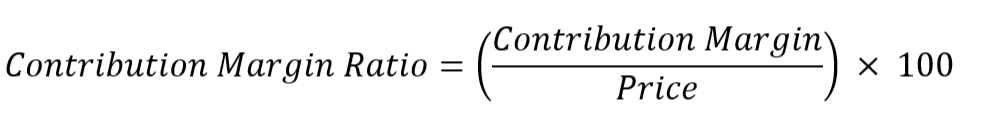Remember
Remember that the contribution margin is the denominator of the breakeven formula, or the price per unit minus the total variable costs per unit.

Once the contribution margin ratio has been determined, it is plugged into the formula below to determine the breakeven point in sales dollars.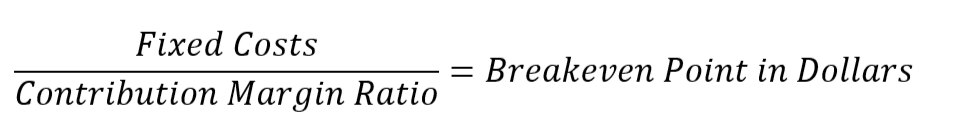## For Example – Calculating a Breakeven Point in Dollars

Thinking back to our online retailer example, let’s calculate the same breakeven point in dollars instead of units. In determining the number of units needed to break even for the month, we calculated the contribution margin to be \$6.53 and we know that the sales price per unit is \$13.99. Plugging those numbers into our contribution margin ratio, we get a result of 46.68 percent.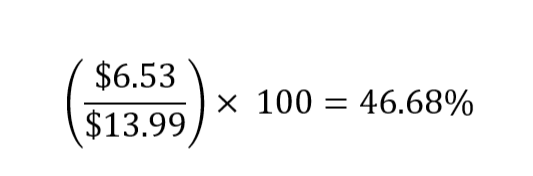The next step is to use our formula for the breakeven point in dollars, which requires the number we just calculated as well as the total fixed costs for the period, which we know come to \$3,980 for the month.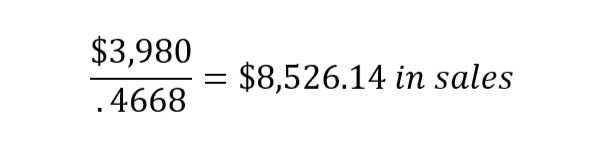Question What if I want to determine the number of sales dollars needed to reach a certain profit threshold in dollars?

If our example online retailer wants to determine the number of sales needed to reach a profit of \$1,200 per month, she will simply add the desired profit to the fixed costs in the equation. By treating the desired profit threshold as a “fixed cost,” the same formula can be applied using the combined total of \$5,180 (known fixed costs of \$3,980 plus the desired profit of \$1,200):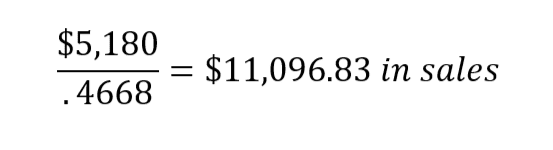### New!Small Business Resources & Tools Page

A one stop shop for entrepreneurs looking for new tools, new strategies, FREE books, and great info. All on one convenient community page.